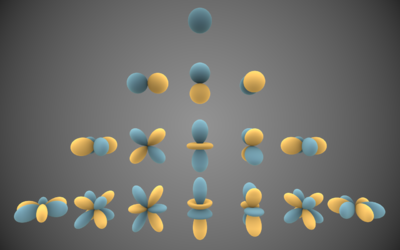This page serves as an entry point to everything related to Spherical Harmonics.

## What are Spherical Harmonics (SH)?Visual representations of the first 4 bands of real spherical harmonic functions. Blue portions are regions where the function is positive, and yellow portions represent regions where it is negative.

According to wikipedia, SH are special functions defined on the surface of a sphere.

In computer graphics, we're using them as a tool to quickly and easily encode or decode a directional information. We use a specific set of spherical harmonics, denoted $Y^l_m(\theta,\phi)$ called Laplace's spherical harmonics.

SH have interesting properties regarding their orthogonality, parity, symmetry and rotation that I will not cover here (cf. the wikipedia page for more info) as this page only is an overview. A very good source of information is Spherical Harmonics Lighting: the Gritty Details by Robin Green that actually covers the practical use of SH for Computer Graphics, it's a well-explained extension of the original work done by Peter Pike Sloane who is pretty much the guy who introduced SH to Computer Graphics for Pre-computed Radiance Transfer (PRT).

I will rather quickly talk about how to construct the SH coefficients and how to encode/decode/convolve signals using SH.

### Constructing the SH coefficients

As can be seen in the inset on the right, Spherical Harmonics are functions defined over the sphere. They are denoted $Y^l_m(\theta,\phi)$ where $l$ is the order of the coefficient. Since SH define harmonic series, there are an infinite amount of possible orders.

For a given order $l$ you have $2l+1$ coefficients selected by the superscript $m\in[-l,+l]$. It ensues that the amount of coefficients for each order is then:

          1           Order 0 - Total = 1 = 1²
2  3  4        Order 1 - Total = 1 + 3 = 4 = 2²
5  6  7  8 9      Order 2 - Total = 1 + 3 + 5 = 9 = 3²
10 11 12 13 14 15 16  Order 3 - Total = 1 + 3 + 5 + 9 = 16 = 4²
$\dots$


We quickly see the amount of coefficients to properly represent each order grows quadratically.

Intuitively, we also can notice that the more coefficients we have, the more "directionalities" we can cover. Order 0 is a constant so it represents the "ambient term", the average response of a signal and has no specific direction. Coefficients for order 1 represent the response to a signal aligned with the X, Y and Z axes. At order 2, we start covering some diagonal directions and higher frequencies.

In fact, if we used an infinite sum of SH coefficients, we could encode or decode a signal perfectly. A great advantage of using SH is that we can recover a partial signal (i.e. a band-limited signal) and we will obtain a low-frequency representation of that signal, as opposed to wavelets that attempt to reconstruct all frequencies of a signal and can show high-frequency noise, a partial SH-encoded signal will always be smooth.

## Archive

You can find an old (2009) explanation about SH Environment Maps.